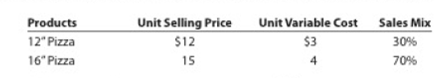# Sales mix and break even sales Data related to the expected sales of two types of frozen pizzas for Norfolk Frozen Foods Inc. for the current year, which is typical of recent years, are as follows: The estimated fixed costs for the current year are $46.800. Instructions 1. Determine the estimated units of sales of the overall (total) product, E, necessary to reach the break-even point for the current year. 2. Based on the break-even sales (units) in part (1), determine the unit sales of both the 12" pizza and 16” pizza for the current year. 3. Assume that the sales mix was 50% 12" pizza and 50% 16" pizza. Compare the break-even point with that in part (1). Why is it so different?BuyFind ### Accounting 27th Edition WARREN + 5 others Publisher: Cengage Learning, ISBN: 9781337272094BuyFind ### Accounting 27th Edition WARREN + 5 others Publisher: Cengage Learning, ISBN: 9781337272094 #### Solutions Chapter Section Chapter 21, Problem 21.5BPR Textbook Problem ## Sales mix and break even salesData related to the expected sales of two types of frozen pizzas for Norfolk Frozen Foods Inc. for the current year, which is typical of recent years, are as follows:The estimated fixed costs for the current year are$46.800.Instructions1. Determine the estimated units of sales of the overall (total) product, E, necessary to reach the break-even point for the current year.2. Based on the break-even sales (units) in part (1), determine the unit sales of both the 12" pizza and 16” pizza for the current year.3. Assume that the sales mix was 50% 12" pizza and 50% 16" pizza. Compare the break-even point with that in part (1). Why is it so different?

Expert Solution

1.

To determine

Sales mix: It refers to the relative distribution of the total sales among the number of products sold by a company. In other words, it is expressed as a percentage of units sold for each product with respect to the total units sold for all the products.

Break-even Point: It refers to a point in the level of operations at which a company experiences its revenues generated is equal to its costs incurred. Thus, when a company reaches at its break-even point, it reports neither an income nor a loss from operations. The formula to calculate the break-even point in sales units is as follows:

Break-evenpointinSales(units) =FixedCostsContributionMarginperunit

To determine: the break-even point in sales units for the overall Product E for the current year.

### Explanation of Solution

Determine the break-even point in sales units for the overall Product E.

Fixed cost =$46,800 Contribution margin per unit =$10.40 per unit (3)

Break-evenpointinSales(units)forProductE] =FixedCostsContributionMarginperunit=$46,800$10.40=4,500units

Working notes:

Note: For break-even analysis, the Product-12” Pizza and Product-13” Pizza are considered as the components of one overall company’s Product E.

Determine the selling price per unit of Product E.

SellingpriceperunitofProductE]=(Sellingpriceperunitof12"Pizza×salesmixof12"Pizza)+(Sellingpriceperunitof16"Pizza×salesmixof16"Pizza)=($12perunit×30%)+($15perunit×70%)=$3.60perunit+$10.50perunit=\$14.10perunit (1)

Determine the variable cost per unit of Product E

Expert Solution

2.

To determine
the break-even sales (units) for Product-12” Pizza and Product-16” Pizza.

Expert Solution

3.

To determine

To compare: the break-even point with that in Part (1).

### Want to see the full answer?

Check out a sample textbook solution.See solution

### Want to see this answer and more?

Bartleby provides explanations to thousands of textbook problems written by our experts, many with advanced degrees!

See solution